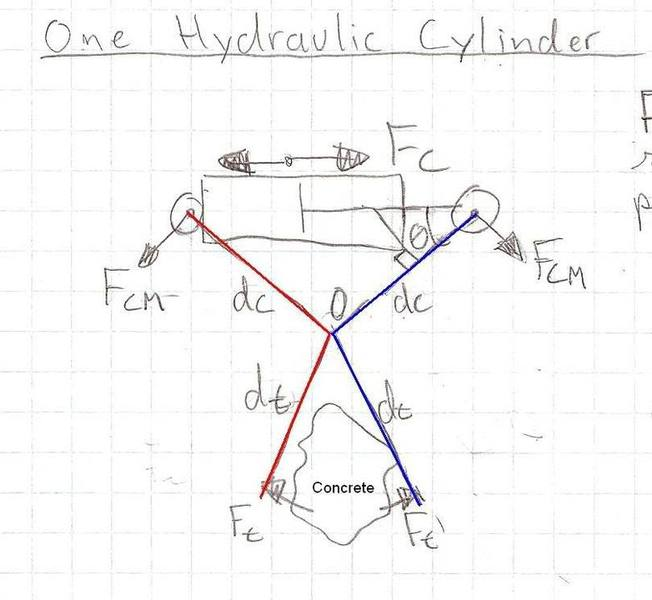# Help with Force calculations

nofx1981
Hi guys,
I need to figure out the crushing force of a concrete crusher. Here's a pic to help illustrate:I have 2 questions:
1) Would the crushing force on the concrete be 2*Ft or only Ft
and
2) Would the force exerted by the cylinder on each jaw be Fc/2 or Fc as shown.

Thanks for you help!

Homework Helper
Hi, nofx1981. Is this for a school assignment?

Studiot
Think about action and reaction and Newtons First Law.
Also think about the principles of levers.

Last edited:
nofx1981
Hi, nofx1981. Is this for a school assignment?

No, this isn't for school. I've finished school a number of years ago.

Think about action and reaction and Newtons First Law.
Also think about the principles of levers.

Yes, I have been thinking of of newton's 1st law and levers and such

My first thought was that the cylinder would exert only half of it's force on each lever and that the crushing force would be the sum of forces at the tips. But now i'm thinking that isn't right.

This is my new train of thought. So the cylinder exerts a force Fc=P*A at an angle of theta on each lever. So there is a moment about O of Fc*sin($$\theta$$)*dc for each lever. These moments will apply a force at the tip of each lever Ft=Fc*sin($$\theta$$)*dc/dt and this is your crushing force, right? Ft=Fcrush?

It's been a while since I've done this kind of stuff, would really just like some confirmation...

Thanks for the help!

Studiot
This is my new train of thought. So the cylinder exerts a force Fc=P*A at an angle of theta on each lever. So there is a moment about O of Fc*sin()*dc for each lever. These moments will apply a force at the tip of each lever Ft=Fc*sin()*dc/dt and this is your crushing force, right? Ft=Fcrush?

This is correct. Otherwise the concrete block would fly off somewhere.
And you worked it out for yourself!

I note Google lists several patents on concrete crushing tongs.